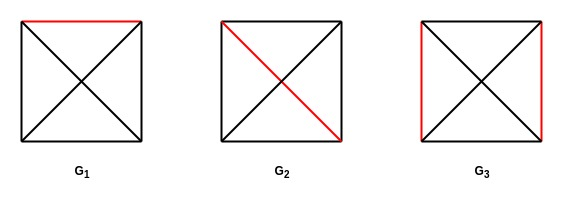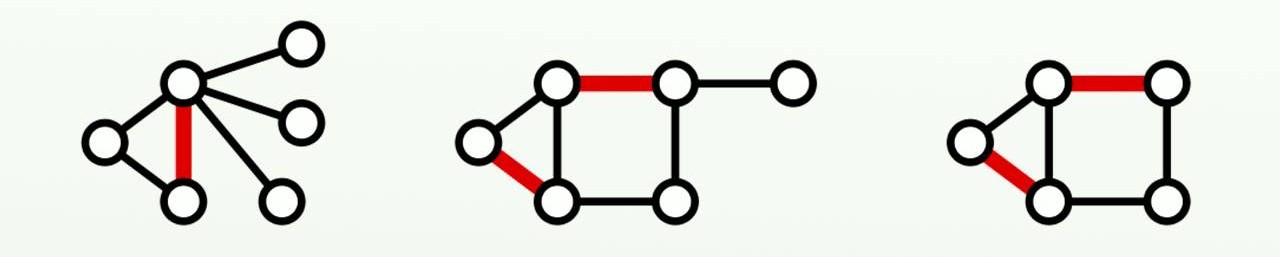# Mathematics | Matching (graph theory)

• Difficulty Level : Medium
• Last Updated : 07 Jan, 2022

Prerequisite – Graph Theory Basics
Given an undirected graph, a matching is a set of edges, such that no two edges share the same vertex. In other words, matching of a graph is a subgraph where each node of the subgraph has either zero or one edge incident to it.
A vertex is said to be matched if an edge is incident to it, free otherwise.
Possible matchings of, here the red edges denote the matching –Matching Terminology –

Maximal Matching – A matchingof graphis said to be maximal if on adding an edge which is inbut not in, makesnot a matching.
In other words, a maximal matchingis not a proper subset of any other matching of. For example, the following graphs are maximal matchings –Adding any edge to any of the above graphs would result in them no longer being a matching.

Maximum Matching – A matchingof graphis said to be maximum if it is maximal and has the maximum number of edges.
There may be many possible maximum matchings of a graph.
Every maximum matching is a maximal matching but not every maximal matching is a maximum matching.
For example, in the first figureis a maximum matching and in the second figure, the second and third graphs are maximum matchings.

Perfect Matching – A matchingof graphis said to be perfect if every vertex is connected to exactly one edge. Every perfect matching is a maximum matching but not every maximum matching is a perfect matching.
Since every vertex has to be included in a perfect matching, the number of edges in the matching must bewhere V is the number of vertices. Therefore, a perfect matching only exists if the number of vertices is even.
For example in the first figure,is a perfect matching.
A matching is said to be near perfect if the number of vertices in the original graph is odd, it is a maximum matching and it leaves out only one vertex. For example in the second figure, the third graph is a near perfect matching.

• Example – Count the number of perfect matchings in a complete graph.
• Solution – If the number of vertices in the complete graph is odd, i.e.is odd, then the number of perfect matchings is 0.
But ifis even then we can derive a general formula for counting the number of perfect matchings.
Sinceis even, we can denote it asfor some positive integer.
Every vertex is connected to every other vertex in a perfect graph, therefore the degree of each vertex is. Out of theseedges we have to choose 1 edge to include two vertices.
We can choose an edge inways. Nowvertices remain andedges remain since the edges connected to the already selected vertices cannot be selected because it is a matching.
So the number of ways of selecting an edge fromedges is.
We can keep choosing edges in the same way, then by product rule-
Number of perfect matchings-.
This can also be written as-So for a perfect graph withvertices the number of perfect matchings is-Bipartite Matching –
Matching has many applications in flow networks, scheduling, and planning, graph coloring, neural networks etc. The most common of these is the scheduling problem where there aretasks which may be completed byworkers. The tasks and workers represent the two sets of vertices in a bipartite graph, where a task is connected to a worker if the worker is able to complete it.
The problem therefore is to find a maximum matching.
More on this topic is discussed in the article – Maximum Bipartite Matching

GATE CS Corner Questions

Practicing the following questions will help you test your knowledge. All questions have been asked in GATE in previous years or in GATE Mock Tests. It is highly recommended that you practice them.

References –

Matching – Wikipedia
Discrete Mathematics and its Applications, by Kenneth H Rosen

This article is contributed by Chirag Manwani. If you like GeeksforGeeks and would like to contribute, you can also write an article using contribute.geeksforgeeks.org or mail your article to contribute@geeksforgeeks.org. See your article appearing on the GeeksforGeeks main page and help other Geeks.

Please write comments if you find anything incorrect, or you want to share more information about the topic discussed above.

My Personal Notes arrow_drop_up
Recommended Articles
Page :